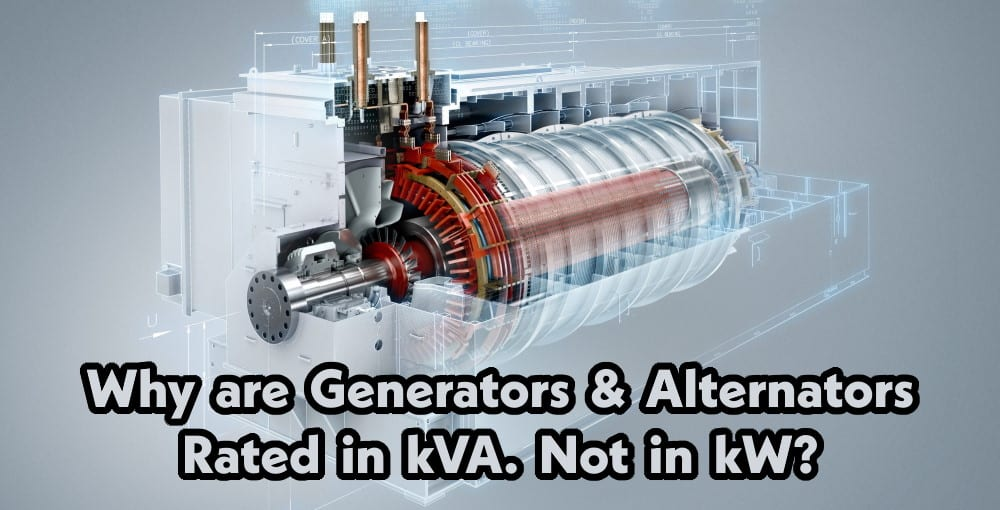# Why Generator & Alternator rated in kVA. Not in kW?

## Why Alternator / Generator  Rated in kVA. Not in kW?

As we already know that why transformer rated in kVA instead of kW, same reason here i.e. the power √3 VL IL Cos θ delivered by the alternator and generator for the same value of current, depends upon p.f. (Power Factor = Cos θ) of the load.

But the alternator conductors are calculated for a definite current and the insulation at magnetic system are designed for a definite voltage independent of p.f. (Cos θ) of the load. For this reason apparent power measured in kVA is regarded as the rated power of the alternator.The main factors manufacturers consider while designing electrical devices and appliances which provide electric power like transformer, UPS, alternators and generators,etc are load and power factor. As they don’t know exactly what is power factor and which kind of load will be connected to the device and appliances.

So they simply design and rate the electrical device according to its maximum current output that the conductors can safely carry while they consider unity power factor (In case of pure resistive load).

If we connect inductive or capasitive load (When power factor is not at least unity), The output would differ than as there are losses occurs due to low power factor.

For this reason, KVA is an apparent power which does not take in to account the PF (Power factor) instead of KW (Real Power).

Where:

KW= KVA X Cos θ

And kVA = KW / Cos θ.

For example,

In case of unity Power factor (1) i.e. pure resistive load. A 100kVA generator or alternator would provide exact 100kW acording to the following formula.

P = V x I x Cos θ

kW = V x I x Cos θ

kW = 100 x 1 = 100 kW

If we put the value of Cos θ as 1, The real power (kW) would be equal to the appearnt power (kVA)

In case of inductive or capacitive load, suppose the power factor is 0.80.

Now the real power would be

kW= KVA X Cos θ

kW = 100 X 0.80

kW= 80 kW.

Now you now that why alternators, generators, transformer and UPS etc are rated in kVA instead of kW.